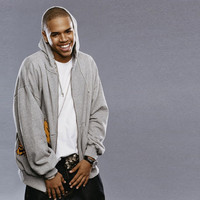música

# Acorde de Zero - Chris Brown```Capo 4

[Verse 1]

Am            G
I thought we were great
Am                       G
You took your love back and ran us off track
Am             G
I counted all the days
Am                              G
'Til you would come back, how stupid was that?

[Pre-Chorus]

F                                     G
Now you're missing what we used to have
Am                   G
Guess the vodka brought the feeling back
Am                 G
I was caught up in the aftermath
F                           Gm
But now, if you really wanna know

[Chorus]

Am             G                          F       G
Ask how many nights I've been thinking of you, zero, zero
Am               G                        F       G
Gave a hundred percent but all I got from you, zero, zero
Am                    G             Am            G
I thought you were the one, then you turned and to run
F                        G
Found somebody better, like I never met ya
Am               G                        F        G
Ask how many nights I've been thinking of you, zero, zero
Am                        G                    F
(Zero, zero, zero, zero, zero, zero, zero, zero, zero, zero, zero)
Gm                           Am
That's how many fucks I give, oh

[Verse 2]

Am                G
Wake up with a dime
Am                    G
Get up to no good, get up and you could
Am              G
Go out, free our minds
F                    Gm
Like you never would, never understood

[Pre-Chorus 2]

F                             G
Now you're missing what we used to have
Am                         G
You been creeping 'round my Instagram
F                          G
I was caught up in the aftermath
F           Gm
But now, if you really wanna know

[Chorus]

Am             G                          F      G
Ask how many nights I've been thinking of you, zero, zero
Am               G                        F       G
Gave a hundred percent but all I got from you, zero, zero
Am                    G             Am              G
I thought you were the one, then you turned and to run
F                        G
Found somebody better, like I never met ya
Am               G                        F        G
Ask how many nights I've been thinking of you, zero, zero
Am                        G                    F
(Zero, zero, zero, zero, zero, zero, zero, zero, zero, zero, zero)
Gm                           Am
That's how many fucks I give

[Bridge]

Am
Broke back then but a nigga rich now
G                                                   F
Got dollars, got euros, got yen, I got pounds, zeros, zeros
Am
G                                                F
My new chick one hundred, and she got her own dinero, zero
Gm
You think I'm thinking 'bout your ass?

[Chorus]

Am             G                          F       G
Ask how many nights I've been thinking of you, zero, zero
Am               G                        F       G
Gave a hundred percent but all I got from you, zero, zero
Am                    G             Am            G
I thought you were the one, then you turned and to run
F                        G
Found somebody better, like I never met ya
Am               G                        F        G
Ask how many nights I've been thinking of you, zero, zero
Am                        G                    F
(Zero, zero, zero, zero, zero, zero, zero, zero, zero, zero, zero)
Gm                           Am
That's how many fucks I give
Am                        G                    F
(Zero, zero, zero, zero, zero, zero, zero, zero, zero, zero, zero)
Gm                         Am
That's how many fucks I give```

Letra subida por: Anónimo

Discos en los que aparece este acorde: Zero (Cd Single) , Royalty , Royalty (Deluxe Edition) , Royalty (Japan Edition)

El acorde de Zero de Chris Brown es una versión de la canción original realizada por colaboradores/usuarios de Coveralia.

¿Has encontrado algún error en esta página? Envíanos tu corrección del acorde

### Zona de usuario

 Recuperar contraseña Regístrate ¿Por qué registrarme?